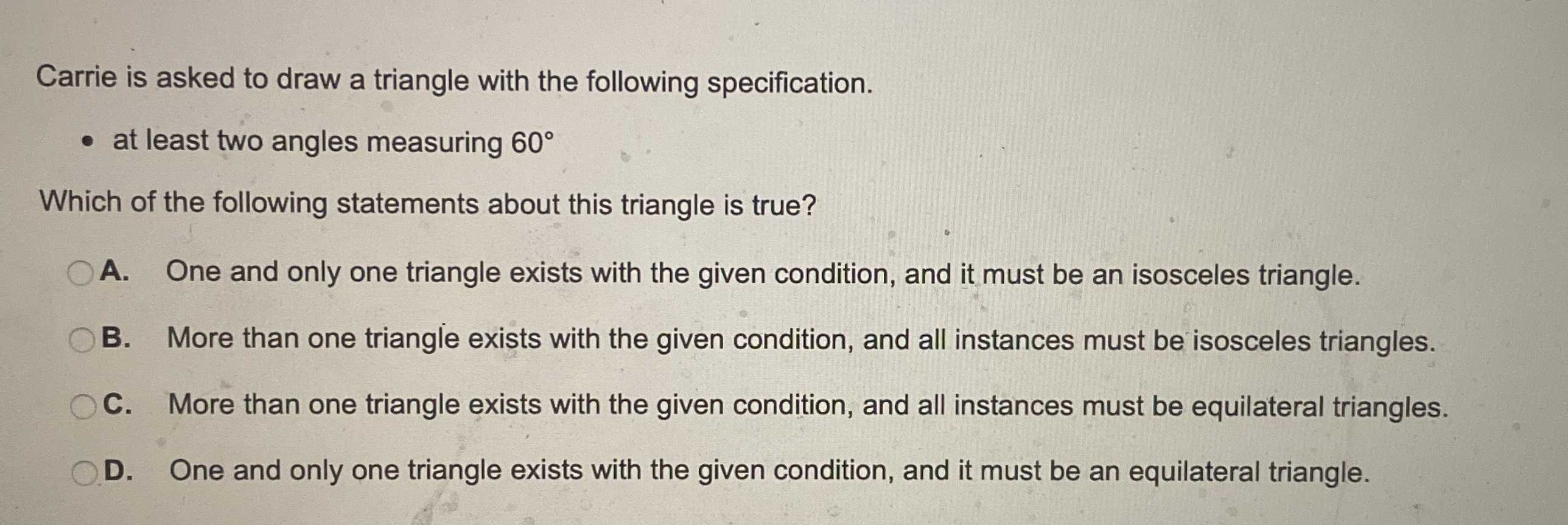### Still have math questions?

Trigonometry
QuestionCarrie is asked to draw a triangle with the following specification.

- at least two angles measuring $$60 ^ { \circ }$$

A. One and only one triangle exists with the given condition, and it must be an isosceles triangle.

B. More than one triangle exists with the given condition, and all instances must be equilateral triangles.

C. More than one triangle exists with the given condition, and all instances must be equilateral triangles.

D. One and only one triangle exists with the given condition, and it must be an equilateral triangle.